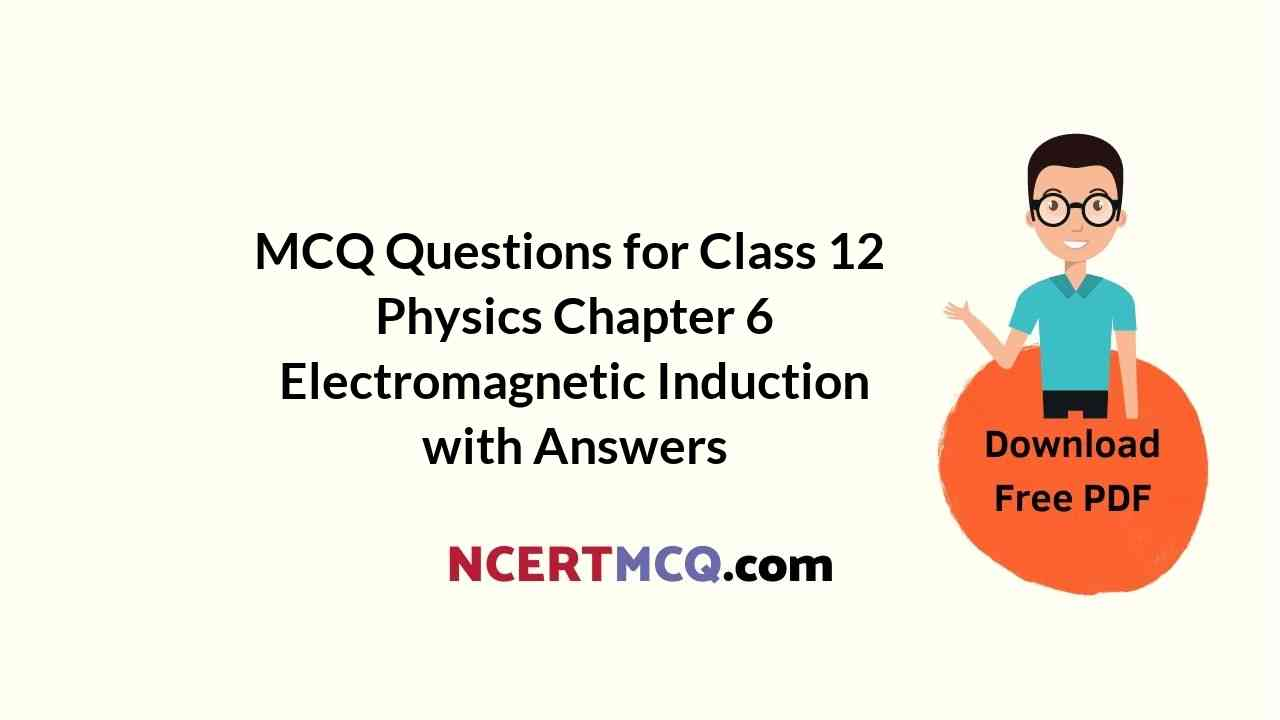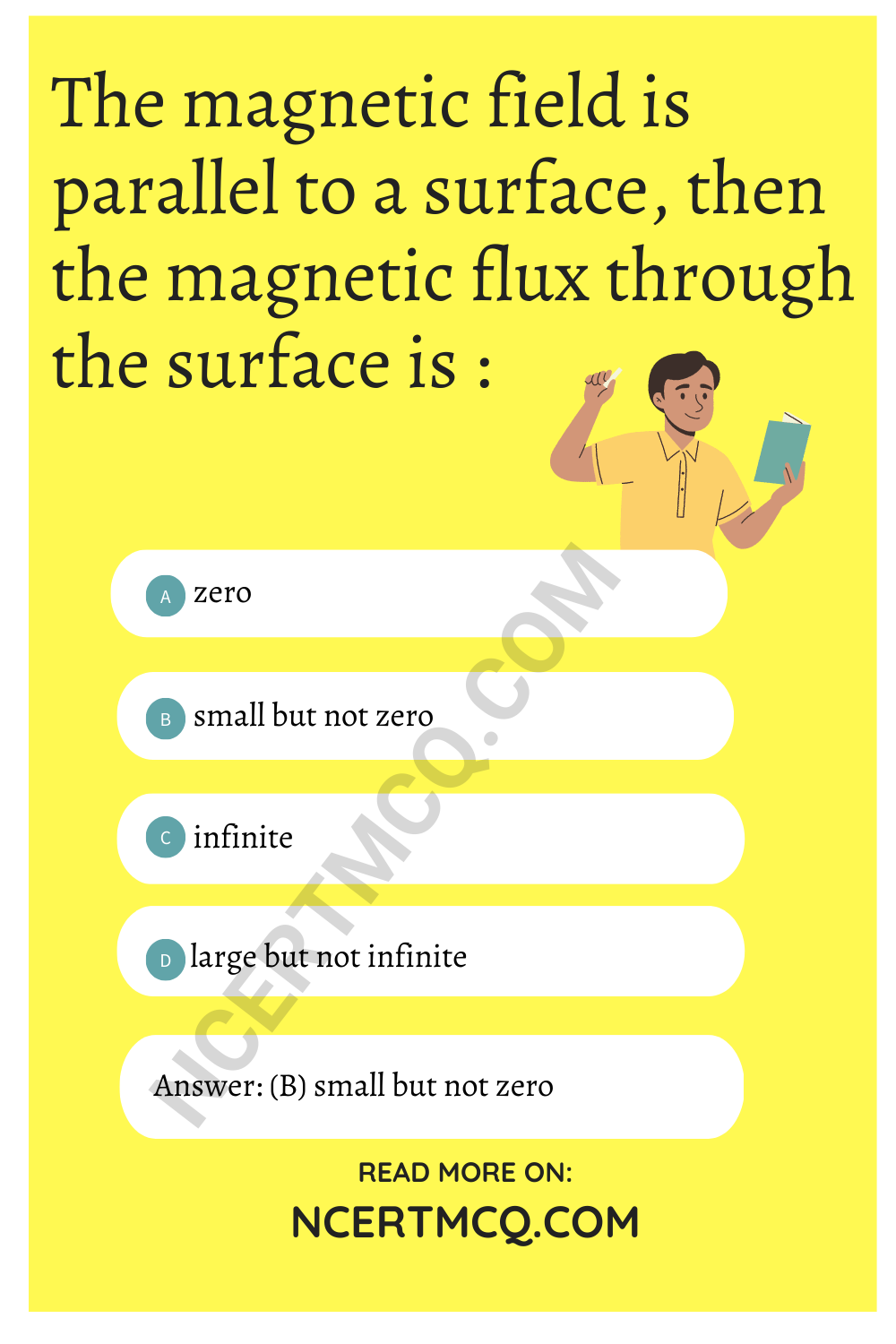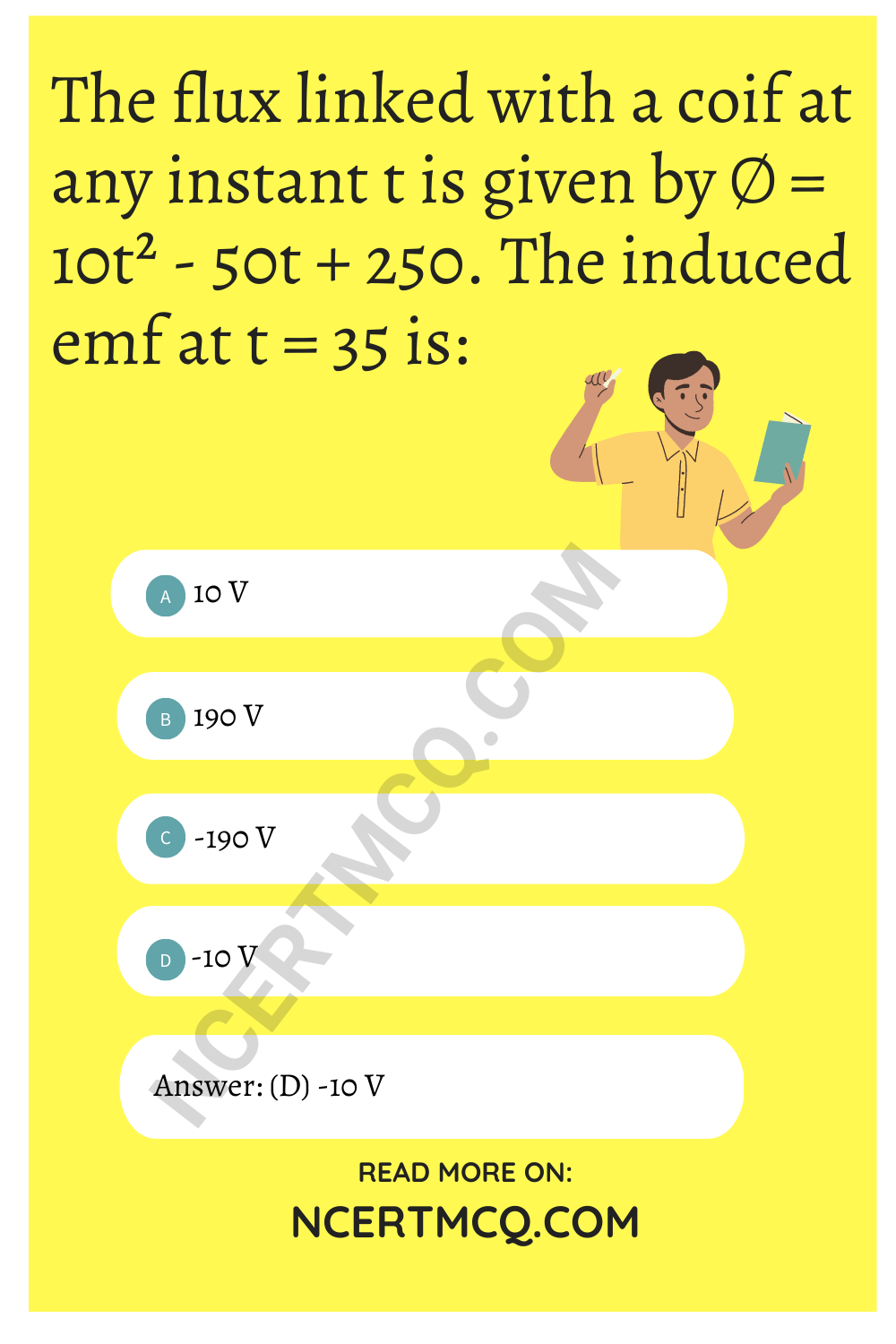Check the below NCERT MCQ Questions for Class 12 Physics Chapter 6 Electromagnetic Induction with Answers Pdf free download. MCQ Questions for Class 12 Physics with Answers were prepared based on the latest exam pattern. We have provided Electromagnetic Induction Class 12 Physics MCQs Questions with Answers to help students understand the concept very well.

## Electromagnetic Induction Class 12 MCQs Questions with Answers

Electromagnetic Induction Class 12 MCQ Question 1.
The coupling co-efficient of the perfectly coupled coils is:
(a) Zero
(b) 1
(c) slightly more than 1
(d) infinite

Class 12 Physics Chapter 6 MCQ Question 2.
1 henry is equal to :
(a) $$\frac{weber}{ampere}$$
(b) $$\frac{weber}{Volt}$$
(c) weber ampere
(d) None of these

Answer: (a) $$\frac{weber}{ampere}$$

Electromagnetic Induction MCQ Question 3.
The magnetic field is parallel to a surface, then the magnetic flux through the surface is :
(a) zero
(b) small but not zero
(c) infinite
(d) large but not infinite

Answer: (b) small but not zeroMCQ On Electromagnetic Induction Class 12 Question 4.
In the expression e = –$$\frac{d∅}{dt}$$, the -ve sign signifies:
(a) The induced emf is produced only when magnetic flux decreases
(b) The induced emf opposes the change in the magnetic flux
(c) The induced emf is opposite to the direction of the flux
(d) None of these

Answer: (b) The induced emf opposes the change in the magnetic flux

Ch 6 Physics Class 12 MCQ Question 5.
An e and a p are moving parallel to each other in a magnetic field. The magnetic force acting on the p+ is:
(a) 1840 times that on e
(b) less than that of e
(c) same as that of e
(d) slightly more than that of e

Answer: (c) same as that of e

Chapter 6 Physics Class 12 MCQ Question 6.
Which of the following is not equal to a henry?
(a) $$\frac{Volt second}{ampere}$$
(b) $$\frac{Volt second^2}{Coulomb}$$
(c) $$\frac{Volt^2 second}{Coulomb}$$
(d) $$\frac{Jole second}{Coulomb^2}$$

Answer: (c) $$\frac{Volt^2 second}{Coulomb}$$

Electromagnetic Induction MCQs Class 12 Question 7.
A transformer is used to light 100 W and 110 V lamp from a 220 V mains. If the main current is 0.5 A. Then the efficiency of the transformer is:
(a) 11%
(b) 50%
(c) 80%
(d) 90%

Physics Class 12 Chapter 6 MCQ Question 8.
The magnetic flux (∅) linked with a coil is related to the number of turns (N) ofthe coil as:
(a) f ∝ N
(b) f ∝ N-1
(c) f ∝ N2
(d) f ∝ N-2

MCQ Of Electromagnetic Induction Question 9.
The magnetic flux (∅) lined with a coil is related to its area (s) as:
(a) ∅ ∝ s
(b) ∅ ∝ s²
(c) ∅ ∝ s1/2
(d) ∅ ∝ s-1/2

Class 12 Physics Chapter 6 MCQ Questions Question 10.
If the magnetic flux linked with a coil through which a current off ampere is set up is ∅, then the coefficient of self-inductance of the coil is:
(a) $$\frac{l}{∅}$$
(b) $$\frac{∅}{l}$$
(c) ∅l
(d) None of these

Answer: (b) $$\frac{∅}{l}$$

Class 12 Physics Ch 6 MCQ Question 11.
In a uniform magnetic field B, a wire in the form of a semicircle of radius r rotated about the diameter of the circle with angular frequency ‘ω’. The axis of rotation is perpendicular to the field. If the total resistance of the circuit is R the mean power generated per period of rotation is :
(a) $$\frac{Bπr^2ω}{2R}$$
(b) $$\frac{(Bπr^2ω)^2}{8R}$$
(c) $$\frac{(Bπrω)^2}{2R}$$
(d) $$\frac{(Bπrω^2)^2}{8R}$$

Answer: (b) $$\frac{(Bπr^2ω)^2}{8R}$$

Physics Chapter 6 Class 12 MCQ Question 12.
The role of inductance is equivalent to:
(a) inertia
(b) force
(c) energy
(d) momentum

MCQ Questions For Class 12 Physics Chapter 6 Question 13.
A metal conductor of length 1 m rotates vertically about one of its ends at angular velocity 5 rad s-1 . If the horizontal component of earth’s magnetism is 2 × 10-5 T, then e.m.f. developed between the two ends of the conductor is:
(a) 5 µV
(b) 50 µV
(c) 5 mV
(d) 50 mV

Electromagnetic Induction MCQs Question 14.
coil of L = 8.4 mH and R = 6 Ω is connected to a 12 V battery. The current in the coil is 1.0 A at approx’time of:
(a) 500 s
(b) 20 s
(c) 35 ms
(d) 1 ms

Class 12 Physics Electromagnetic Induction MCQ Question 15.
If I current is flowing inductance L, then the dimension of $$\frac{3}{2}$$ LI² is equivalent to:
(a) charge
(b) force
(c) momentum
(d) energy

Electromagnetic Induction MCQ Class 12 Question 16.
The phase difference between the flux linkage and the emf in a rotating coil in a uniform magnetic field is:
(a) zero
(b) $$\frac{π}{2}$$
(c) $$\frac{π}{4}$$
(d) π

Answer: (c) $$\frac{π}{4}$$

Class 12 Electromagnetic Induction MCQ Question 17.
A choke is used as a resistance in :
(a) dc circuits
(b) ac circuits
(c) both ac and dc circuits
(d) neither (a) nor (b)

Chapter 6 Class 12 Physics MCQ Question 18.
The SI unit of magnetic flux is:
(a) T
(b) Tn-2
(c) Wb
(d) Wb m-2

MCQ Questions On Electromagnetic Induction Class 12 Question 19.
For purely capacitive circuits, power factor is:
(a) 0
(b) -1
(c) 1
(d) infinity

MCQ Of Chapter 6 Physics Class 12 Question 20.
The magnetic flux linked with a coil is inversely proportional to the?
(a) magnetic field
(b) area of cross section
(c) number of turns
(d) none of these

When the magnetic field is parallel to a surface, then the magnetic flux through the surface is:
(a) infinite
(b) zero
(c) small but not zero
(d) large but not infinite.

Question 22.
The energy stored in a 50 mH inductor carrying a current of 4 A is:
(a) 0.1 J
(b) 0.4 J
(c) 0.04 J
(d) 0.01 J

Question 23.
The flux linked with a coif at any instant t is given by ∅ = 10t² – 50t + 250. The induced emf at t = 35 is:
(a) 10 V
(b) 190 V
(c) -190 V
(d) -10 VQuestion 24.
Two coils of self inductance 2 mil and 8 mil are placed so close together that the effective flux in one coil is completely linked with other. The mutual inductance between these coils is :
(a) 4 mH
(b) 16 mH
(c) 10 mH
(d) 6 mH

Question 25.
The core of a transformer is laminated because:
(a) rusting of core may be prevented
(b) ratio of voltage in primary and secondary may be increased.
(c) energy losses due to eddy current may be minimised
(d) The weight of transformer may be reduced